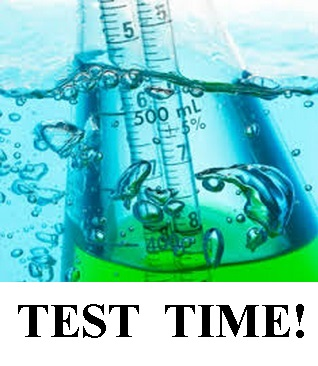# Chemical Reaction Test 2

6 Questions | Total Attempts: 321SettingsReactions in Aqueous Solution, Gases, Liquids and Intermolecular Forces

Related Topics
• 1.
• A.

A

• B.

B

• C.

C

• D.

D

• 2.
Consider 100.0 mL of a 1.0 M solution of NaCl in a beaker. After several days, you test the solution and find that it has a concentration of 1.33 M. How much water must have evaporation?
• A.

20.0 mL

• B.

25.0 mL

• C.

75.0 mL

• D.

80.0 mL

• 3.
• A.

The observed pressure will be less than the ideal pressure, and the volume available for the gas particles is less than the volume of the container.

• B.

The observed pressure will be less than the ideal pressure, and the volume available for the gas particles is greater than the volume of the container.

• C.

The observed pressure will be greater than the ideal pressure, and the volume available for the gas particles is greater than the volume of the container.

• D.

The observed pressure will be greater than the ideal pressure, and the volume available for thee gas particles is less than the volume of the container.

• 4.
A sample of neon gas is contained in the left hand bulb and a sample of helium gas is contained in the right hand bulb of a two bulb container connected by a valve, as shown in the diagram. Initially the valve is closed.The left hand bulb has a volume of 9.00 L, and the Ne gas is at a pressure of 2.00 atm. The right hand bulb has a volume of 3.00L, and the He gas is at a pressure of 4.00 atm. After the valve is opened, what is true about the relative partial pressures of helium and neon? Assume constant temperature.
• A.

The partial pressure of helium is 1.50 times as great as the partial pressure of neon

• B.

The partial pressure of neon is 2.00 times as great as the partial pressure of helium

• C.

The partial pressure of neon is 1.50 times as great as the partial pressure of helium

• D.

The partial pressure of helium is 2.00 times as great as the partial pressure of neon

• 5.
The boiling points of hydrogen chloride and hydrogen bromide are given in the table below. Based on periodicity and intermolecular forces, which of the other two hydrogen halides would have a boiling point that is higher than that of HBr?CompoundHClHBrHiHFBoiling Point (degrees celsius)-85-66??
• A.

HI Only

• B.

HF Only

• C.

HI and HF

• D.

Neither HI nor HF

• 6.
Based on molecular structure and intermolecular forces, which of these substances would you predict to have the greatest viscosity?(Choose carefully)
• A.

A

• B.

B

• C.

C

• D.

D